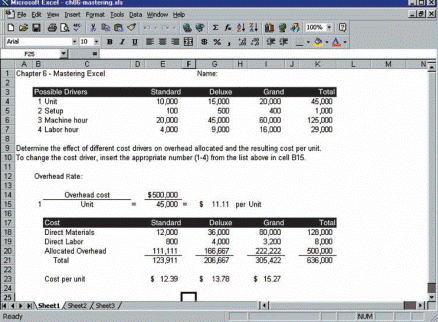### Create an Account

Home / Questions / Beasley Company makes three types of exercise machines Data have been accumulated for fou...

# Beasley Company makes three types of exercise machines Data have been accumulated for four possible overhead drivers Data for these four possible drivers are shown in rows 3 to 7 of the following

Beasley Company makes three types of exercise machines. Data have been accumulated for four possible overhead drivers. Data for these four possible drivers are shown in rows 3 to 7 of the following spreadsheet.

Required

Construct a spreadsheet that will allocate overhead and calculate unit cost for each of these alternative drivers. A screen capture of the spreadsheet and data follows.1. This spreadsheet uses a function called vertical lookup. This function can pull the appropriate values from a table. The form of this function is = VLOOKUP (value, table, column#). In this example, the table is in cells B4 to K7. Three examples of the use of VLOOKUP follow.

2. Cell C15 is =VLOOKUP (B15, B4.K7, 2). This function operates by using the one (1) in cell B15 to look up a value in the table. Notice that the table is defined as B4.K7 and that the function is looking up the value in the second column, which is Unit.

3. Cell E15 is = VLOOKUP (B15, B4.K7, 10). In this case, the function is looking up the value in the tenth column, which is 45,000. Be sure to count empty columns.

4. Cell E20 is = VLOOKUP (B15, B4.K7, 4)*G15. In this case, the function is looking up the value in the fourth column, which is \$10,000. Be sure to count empty columns.

5. Cells I15, G20, and I20 also use the VLOOKUP function.

6. After completing the spreadsheet, you can change the value in cell B15 (1-4) to see the effect of choosing a different driver for overhead.

To this point we have assumed the Magnificent Modems produced only one type of modem. Suppose instead we assume the company produces several different kinds of modems. The production process differs for each type of product. Some require more setup time than others, they are produced in different batch sizes, and they require different amounts of indirect labor (supervision). Packaging and delivery to customers also differs for each type of modem. Even so, Magnificent Modems uses a single allocation base (number of units) to allocate overhead costs.

Required

Write a brief memo that explains how Magnificent Modems could benefit from an ABC cost system.

Jun 30 2020 View more View LessSubscribe To Get Solution